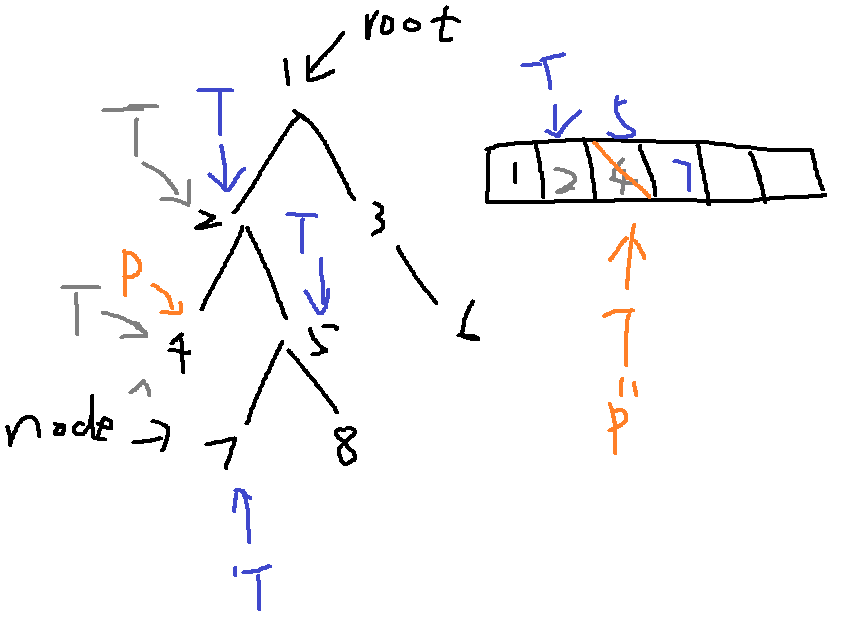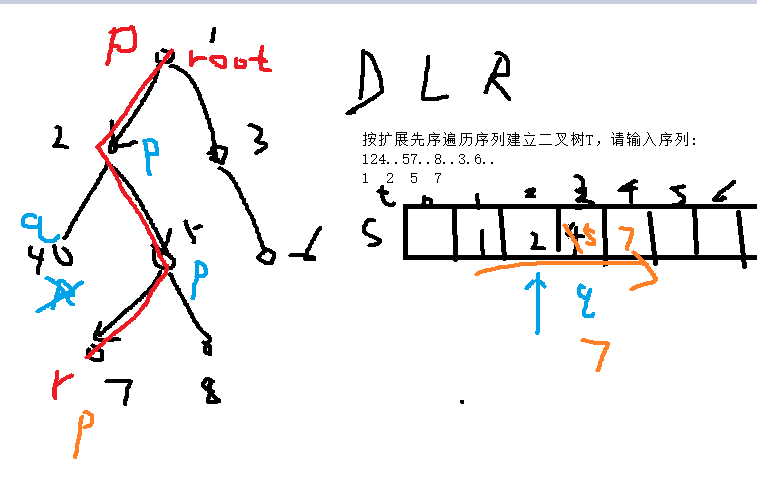# 路径

``````bool path(BiTNode* root, BiTNode* node, Stack* s);
``````

``````typedef int DataType;

typedef struct Node{
DataType data;
struct Node* left;
struct Node* right;
}BiTNode, *BiTree;
``````

``````#define Stack_Size 50
typedef BiTNode* ElemType;
typedef struct{
ElemType elem[Stack_Size];
int top;
}Stack;

void init_stack(Stack *S); // 初始化栈
bool push(Stack* S, ElemType x); //x 入栈
bool pop(Stack* S, ElemType *px); //出栈，元素保存到px所指的单元，函数返回true,栈为空时返回 false
bool top(Stack* S, ElemType *px); //获取栈顶元素，将其保存到px所指的单元，函数返回true，栈满时返回 false
bool is_empty(Stack* S);  // 栈为空时返回 true，否则返回 false
``````

``````#include <stdlib.h>
#include <stdio.h>
#include "bitree.h" //请不要删除，否则检查不通过

bool path(BiTNode* root, BiTNode* node, Stack* s){

}``````

``````bool path(BiTNode* root, BiTNode* node, Stack* s)
{
BiTree T = root, p=NULL;
if (T == NULL || node == NULL || !is_empty(s))
return false;
while (T || !is_empty(s)) {
while (T) {
push(s, T);
if (T == node)
return true;
T = T->left;
}
top(s, &T);
if (!T->right || T->right == p) {
p = T;
pop(s, &T);
T = NULL;
} else
T = T->right;
}
return false;
}
``````

``````#include <stdio.h>
#include <malloc.h>
#include <conio.h>

typedef char DataType;

typedef struct Node
{
DataType data;
struct Node* LChild;
struct Node* RChild;
}BiTNode, * BiTree;

void CreateBiTree(BiTree* bt)
{
char ch;
ch = getchar();
if (ch == '.') *bt = NULL;
else
{
*bt = (BiTree)malloc(sizeof(BiTNode)); //生成一个新结点
(*bt)->data = ch;
CreateBiTree(&((*bt)->LChild)); //生成左子树
CreateBiTree(&((*bt)->RChild)); //生成右子树
}
}

#define  NUM  20

void path(BiTree root, BiTNode* r)
{
BiTNode* p, * q;
int i, find = 0, top = 0;
BiTNode* s[NUM];
q = NULL;   /* 用q保存刚遍历过的结点 */
p = root;
while ((p != NULL || top != 0) && !find)
{
while (p != NULL)
{
top++;
s[top] = p;
p = p->LChild;
}                /* 遍历左子树 */
if (top > 0)
{
p = s[top];       /* 根结点 */
if (p->RChild == NULL || p->RChild == q)
{
if (p == r)   /*找到r所指结点，则显示从根结点到r所指结点之间的路径*/
{
for (i = 1; i <= top; i++)
printf("%c  ", s[i]->data);
find = 1;
}
else
{
q = p;        /* 用q保存刚遍历过的结点 */
top--;
p = NULL;    /* 跳过前面左遍历，继续退栈 */
}
}
else
p = p->RChild;     /* 遍历右子树 */
}
}
}
/*
void path(BiTree root, char r)
{
BiTNode  *p, *q;
int i, find=0, top=0;
BiTNode *s[NUM];
q = NULL;   / * 用q保存刚遍历过的结点 * /
p = root;
while ( (p != NULL || top != 0) && !find )
{
while ( p != NULL )
{
top++;
s[top] = p;
p = p->LChild;
}                / * 遍历左子树 * /
if (top > 0)
{
p=s[top];       / * 根结点 * /
if ( p->RChild == NULL || p->RChild == q )
{
if(p->data == r)   / *找到r所指结点，则显示从根结点到r所指结点之间的路径* /
{
for(i=1;i<=top;i++)
printf("%c  ",s[i]->data);
find=1;
}
else
{
q = p;        / * 用q保存刚遍历过的结点 * /
top--;
p = NULL;    / * 跳过前面左遍历，继续退栈 * /
}
}
else
p = p->RChild;     / * 遍历右子树 * /
}
}
}*/

void main()
{
BiTree T;
BiTNode* p;
//	char c;
printf("按扩展先序遍历序列建立二叉树T，请输入序列:\n");
CreateBiTree(&T);
/*
printf("请输入一个字符:");
fflush(stdin);
scanf("%c",&c);*/
//	path(T,c);
p = T->LChild->RChild->LChild;
path(T, p);
getch();
}``````

### 解析：求从根结点到某结点间的路径

``````按扩展先序遍历序列建立二叉树T，请输入序列:
124..57..8..3.6..
1  2  5  7``````### SpringBoot框架_流星...的博客-程序员宝宝

SpringBoot框架一、SpringBoot简介1.1 SpringBoot是什么1.2 SpringBoot的特点1.3 SpringBoot的优缺点二、SpringBoot的使用2.1 创建SpringBoot项目2.2 SpringBoot的配置文件2.2.1 pom文件2.2.2 application.properties2.2.2 application.yml3.4 Spring...

### 【GDOI2018模拟7.10】C_Jacky35的博客-程序员宝宝_【gdoi2018模拟7.10】c

DescriptionInputOutput一行表示答案Sample Inputaa abSample Output2Solution这题直接递归暴力就行了 设暴力带3个参数x,y,l表示上面到x，下面到y，匹配长度为l 预处理一些东西，比如上面第x个字符匹配下面第y个字符之后的第一个是哪个等 加记忆化 就可以过了Code#include<cstdio>#include<cstring>

### axios参考封装_wahaha-me的博客-程序员宝宝

http。js/**axios封装 * 请求拦截、相应拦截、错误统一处理 */import axios from 'axios';import QS from 'qs';// import { Toast } from 'vant';import { Message } from "element-ui";import store from '../store/index'//...

### linux内核编译最详细,Linux内核编译详细教程，linux内核编译_weixin_39969340的博客-程序员宝宝

Linux内核编译详细教程，linux内核编译尝试编译下Linux-kernel 4.14.14，使用Ubuntu 16.04 64位 系统。kernel-4.14.14 内核文件约96MB，解压后得到linux-4.14.14目录约900MB。在终端中切换到解压后的linux-4.14.14文件目录，执行下面的命令：1. .config复制一份当前系统编译时的配置，在/usr/src目录下\$ l...

### pcm alaw java_查表法实现PCM与Alaw、μlaw之间的格式转换_weixin_39830917的博客-程序员宝宝

static byte ALawCompressTable[] ={1, 1, 2, 2, 3, 3, 3, 3, 4, 4, 4, 4, 4, 4, 4, 4,5, 5, 5, 5, 5, 5, 5, 5, 5, 5, 5, 5, 5, 5, 5, 5,6, 6, 6, 6, 6, 6, 6, 6, 6, 6, 6, 6, 6, 6, 6, 6,6, 6, 6, 6, 6, 6, 6, 6, 6...

### junit 5 + mockito 编写单元测试_junit5 mockito @resource_云端小飞熊的博客-程序员宝宝

junit 5 + mockito 编写单元测试最近在学习过程中，涉及到了单元测试的小情况，一下就是单元测试的各个层之间的单元测试，一开始只用junit5做测试，后面发现测试的用例涉及到了数据库，不方便合作开发，故此引进mockito如有不当之处，欢迎指正：.dao层：@ExtendWith(SpringExtension.class)@SpringBootTestclass OrganizationMapperTest { @Mock OrganizationMapper organiz